﻿ 5. Detailed description of the Actions > 5.10. TA - GIS - Geographical Information System > 5.10.10. Reproject GIS

# 5.10.10. Reproject GIS

Icon: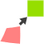Function: reprojectGIS

Property window: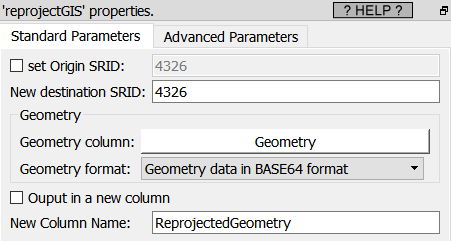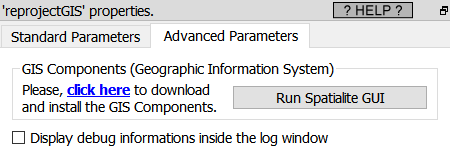Short description:

Reproject geometries Inside a new coordinate system

Long Description:

Most of the visualization Actions inside Anatella do require to get their geometries described in decimal degrees (i.e. inside the coordinate system with the 4326 SRID).

One particularity of the “SRID=4326 coordinate system” is that a “step” of 10 degree of longitude (i.e. along the direction of the “X” axis) represents 354 Km at the equator (i.e. when the latitude is zero) and the same “step” of 10 degree of longitude near the Noth Pole (i.e. when the latitude is near 90°) is nearly zero Km. All this is explained in the following illustrations: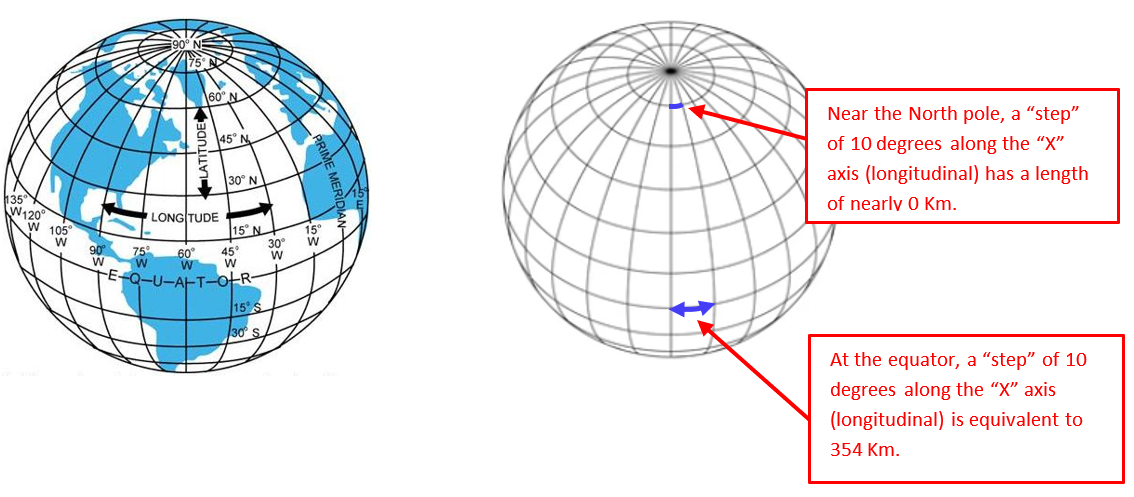In other words, inside the 4326 SRID, a step of one unit along the X axis does not always “moves us” of the same distance (it depends of the value of the Y axis). Hopefully, in the direction of the Y axis –  the latitude – all the steps have the same contant length. So, moving in the X direction (i.e. changing longitude) and moving on the Y direction (i.e. changing latitude) is definively not the same. Here is an example of this strange phenomenon: We created some shapes that looks like perfect circles inside the “SRID=4326 coordinate system”: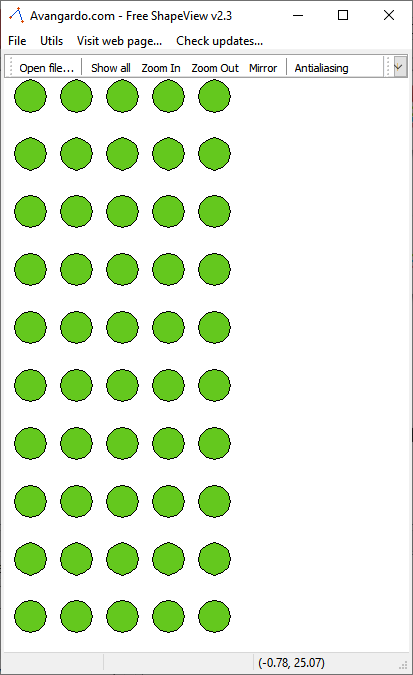…but when we look at these same “perfect circles” on the Earth spheroid, we actually see: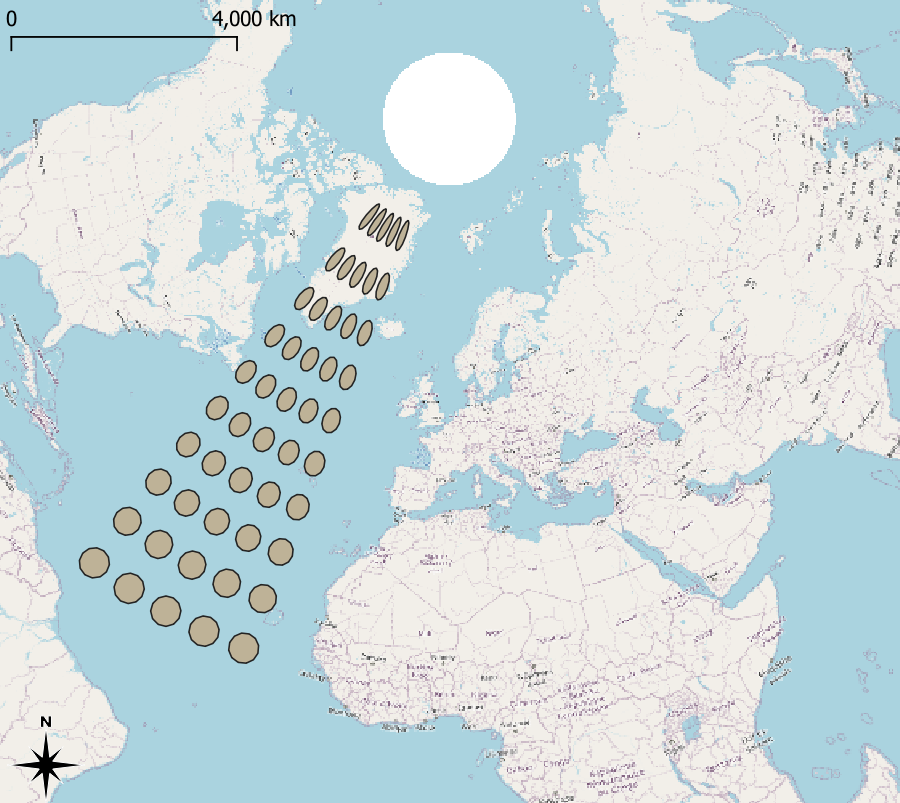This example illustrates very well that the “X axis” (i.e. the longitude direction) “shrinks” when we approach the poles.

Many complex GIS Actions (such as these Actions: KNN, ConcaveHull, SimplifyGIS, ExpandGIS, TesselateGIS) do not work very well inside this “SRID=4326 coordinate system” because these Actions all assume that we are in a standard planar cartesian coordinate system (they totally ignore the fact that our geometries were first defined on a spheroid): i.e. doing a “step” of one unit-length always “move us” of the same constant distance whatever the direction of the step. Hopefully there is a solution to this problem: re-projection! The Anatella Reproject action allows to take the geometries defined inside the problematic 4326 and reproject them inside a new coordinates system that has the nice “planar&cartesian” properties (i.e. all directions are equivalent). Once inside this new coordinate system, you can thus safely use all the complex GIS Actions (KNN, ConcaveHull, SimplifyGIS, ExpandGIS, TesselateGIS) to do all your computations. Also, typically, at the end of all the computations, you’ll reproject inside the 4326 SRID, just for visualization purposes (because, most of the time, the visualizations require the 4326 SRID).